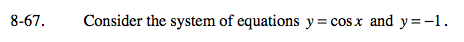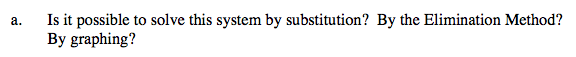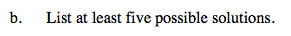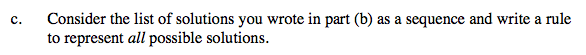Home > A2C > Chapter 8 > Lesson 8.1.4 > Problem8-67

8-67.
1. Consider the system of equations y = cos x and y = −1. 8-67 HW eTool (Desmos). Homework Help ✎

1. Is it possible to solve this system by substitution? By the Elimination Method? By graphing?

2. List at least five possible solutions.

3. Consider the list of solutions you wrote in part (b) as a sequence and write a rule to represent all possible solutions.Use substitution: cos(x) = −1. Solve for x.List five values where the graphs intersect.List your values from part (b) in order and look for a relationship.

x = (−180 + 360n)° for all integers n

Use the eTool below to help answer parts (a) and (b).
Click the link at right for the full version of the eTool: A2C 8-67 HW eTool